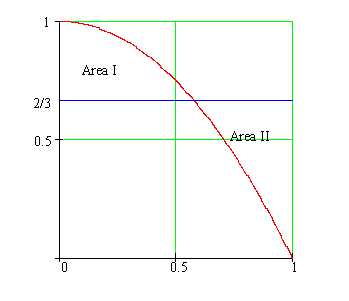Calculus on Demand at Dartmouth College Lecture 11 | Index | Lecture 13 Lecture 12Resources

Math 3 Course Syllabus
Practice Exams

Contents

In this lecture we study something called the "Mean Value Theorem." This theorem allows us to take an "at-a-point" concept like the derivative and use it to study the global behavior of a function. We will also learn how to differentiate a function for which we do not have an explicit formula.

Quick Question

Suppose Area I (above the blue line and below the parabola) equals Area II (above the parabola and below the blue line). What is the total area enclosed by the parabola?Outline

Outlines for
The Mean Value Theorem
Implicit Differentiation

Textbook

The Mean Value Theorem
Implicit Differentiation